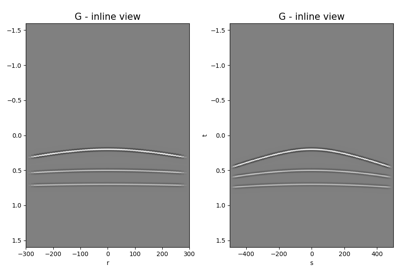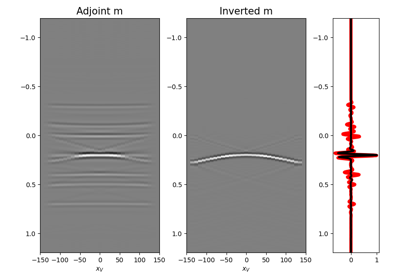# pylops.waveeqprocessing.MDC¶

pylops.waveeqprocessing.MDC(G, nt, nv, dt=1.0, dr=1.0, twosided=True, fast=None, dtype=None, fftengine='numpy', transpose=True, saveGt=True, conj=False, usematmul=False, prescaled=False)[source]

Multi-dimensional convolution.

Apply multi-dimensional convolution between two datasets. If transpose=True, model and data should be provided after flattening 2- or 3-dimensional arrays of size $$[n_r \;(\times n_{vs}) \times n_t]$$ and $$[n_s \;(\times n_{vs}) \times n_t]$$ (or $$2n_t-1$$ for twosided=True), respectively. If transpose=False, model and data should be provided after flattening 2- or 3-dimensional arrays of size $$[n_t \times n_r \;(\times n_{vs})]$$ and $$[n_t \times n_s \;(\times n_{vs})]$$ (or $$2n_t-1$$ for twosided=True), respectively.

Warning

A new implementation of MDC is provided in v1.5.0. This currently affects only the inner working of the operator and end-users can use the operator in the same way as they used to do with the previous one. Nevertheless, it is now reccomended to use the operator with transpose=False, as this behaviour will become default in version v2.0.0 and the behaviour with transpose=True will be deprecated.

Parameters: G : numpy.ndarray Multi-dimensional convolution kernel in frequency domain of size $$[n_s \times n_r \times n_{f_\text{max}}]$$ if transpose=True or size $$[n_{f_\text{max}} \times n_s \times n_r]$$ if transpose=False nt : int Number of samples along time axis for model and data (note that this must be equal to $$2n_t-1$$ when working with twosided=True. nv : int Number of samples along virtual source axis dt : float, optional Sampling of time integration axis $$\Delta t$$ dr : float, optional Sampling of receiver integration axis $$\Delta r$$ twosided : bool, optional MDC operator has both negative and positive time (True) or only positive (False) fast : bool, optional Deprecated, will be removed in v2.0.0 dtype : str, optional Deprecated, will be removed in v2.0.0 fftengine : str, optional Engine used for fft computation (numpy, scipy or fftw) transpose : bool, optional Transpose G and inputs such that time/frequency is placed in first dimension. This allows back-compatibility with v1.4.0 and older but will be removed in v2.0.0 where time/frequency axis will be required to be in first dimension for efficiency reasons. saveGt : bool, optional Save G and G.H to speed up the computation of adjoint of pylops.signalprocessing.Fredholm1 (True) or create G.H on-the-fly (False) Note that saveGt=True will be faster but double the amount of required memory conj : str, optional Perform Fredholm integral computation with complex conjugate of G usematmul : bool, optional Use numpy.matmul (True) or for-loop with numpy.dot (False) in pylops.signalprocessing.Fredholm1 operator. Refer to Fredholm1 documentation for details. prescaled : bool, optional Apply scaling to kernel (False) or not (False) when performing spatial and temporal summations. In case prescaled=True, the kernel is assumed to have been pre-scaled when passed to the MDC routine. ValueError If nt is even and twosided=True

MDD
Multi-dimensional deconvolution

Notes

The so-called multi-dimensional convolution (MDC) is a chained operator . It is composed of a forward Fourier transform, a multi-dimensional integration, and an inverse Fourier transform:

$y(t, s, v) = \mathscr{F}^{-1} \Big( \int_S G(f, s, r) \mathscr{F}(x(t, r, v))\,\mathrm{d}r \Big)$

which is discretized as follows:

$y(t, s, v) = \sqrt{n_t} \Delta t \Delta r\mathscr{F}^{-1} \Big( \sum_{i_r=0}^{n_r} G(f, s, i_r) \mathscr{F}(x(t, i_r, v)) \Big)$

where $$\sqrt{n_t} \Delta t \Delta r$$ is not applied if prescaled=True.

This operation can be discretized and performed by means of a linear operator

$\mathbf{D}= \mathbf{F}^H \mathbf{G} \mathbf{F}$

where $$\mathbf{F}$$ is the Fourier transform applied along the time axis and $$\mathbf{G}$$ is the multi-dimensional convolution kernel.

  Wapenaar, K., van der Neut, J., Ruigrok, E., Draganov, D., Hunziker, J., Slob, E., Thorbecke, J., and Snieder, R., “Seismic interferometry by crosscorrelation and by multi-dimensional deconvolution: a systematic comparison”, Geophysical Journal International, vol. 185, pp. 1335-1364. 2011.

## Examples using pylops.waveeqprocessing.MDC¶Multi-Dimensional Convolution09. Multi-Dimensional Deconvolution